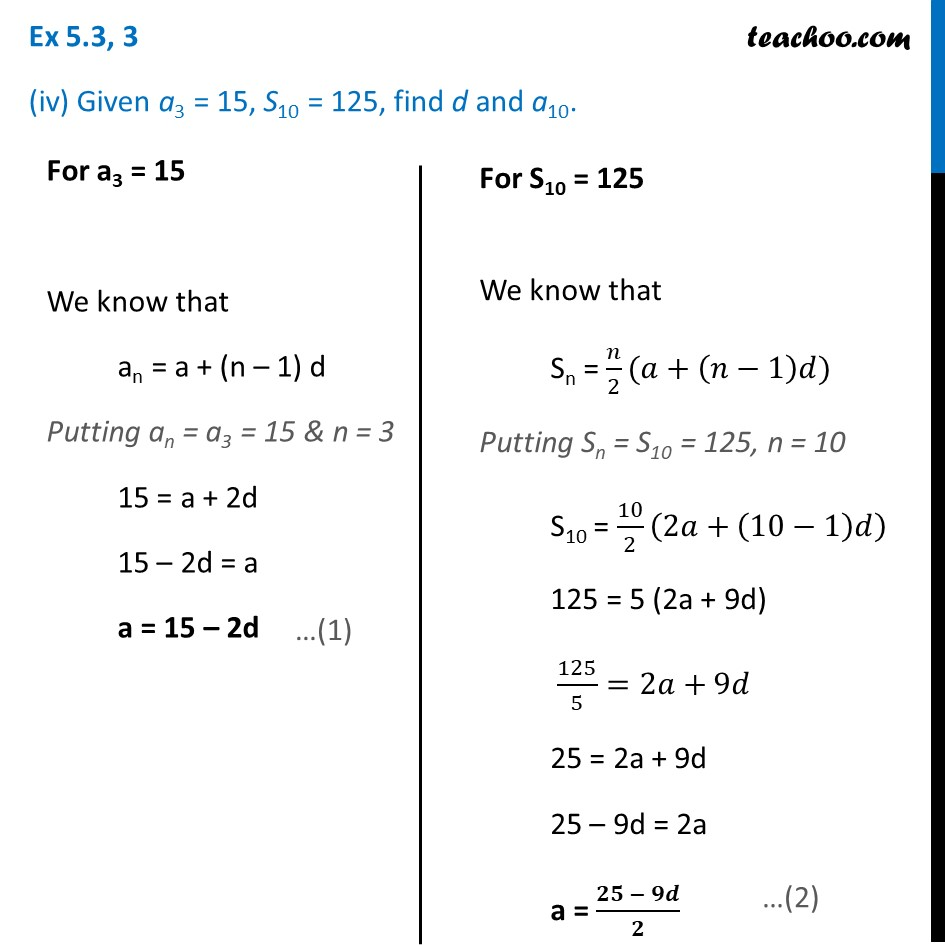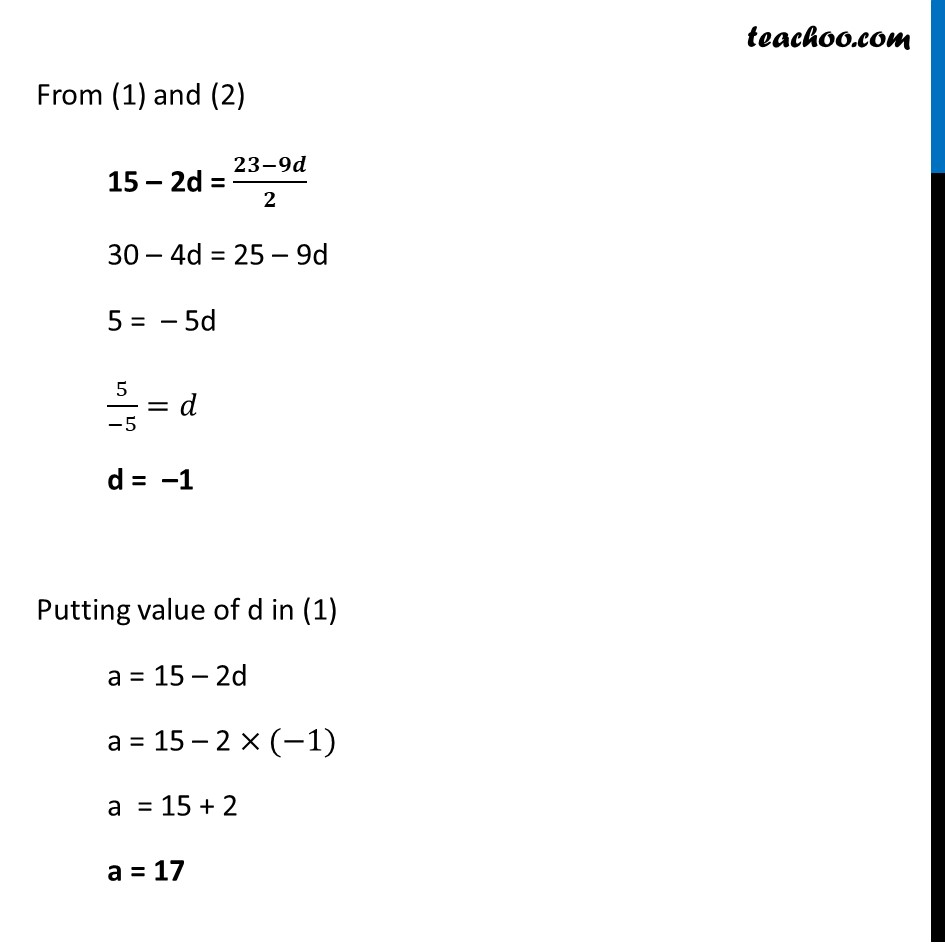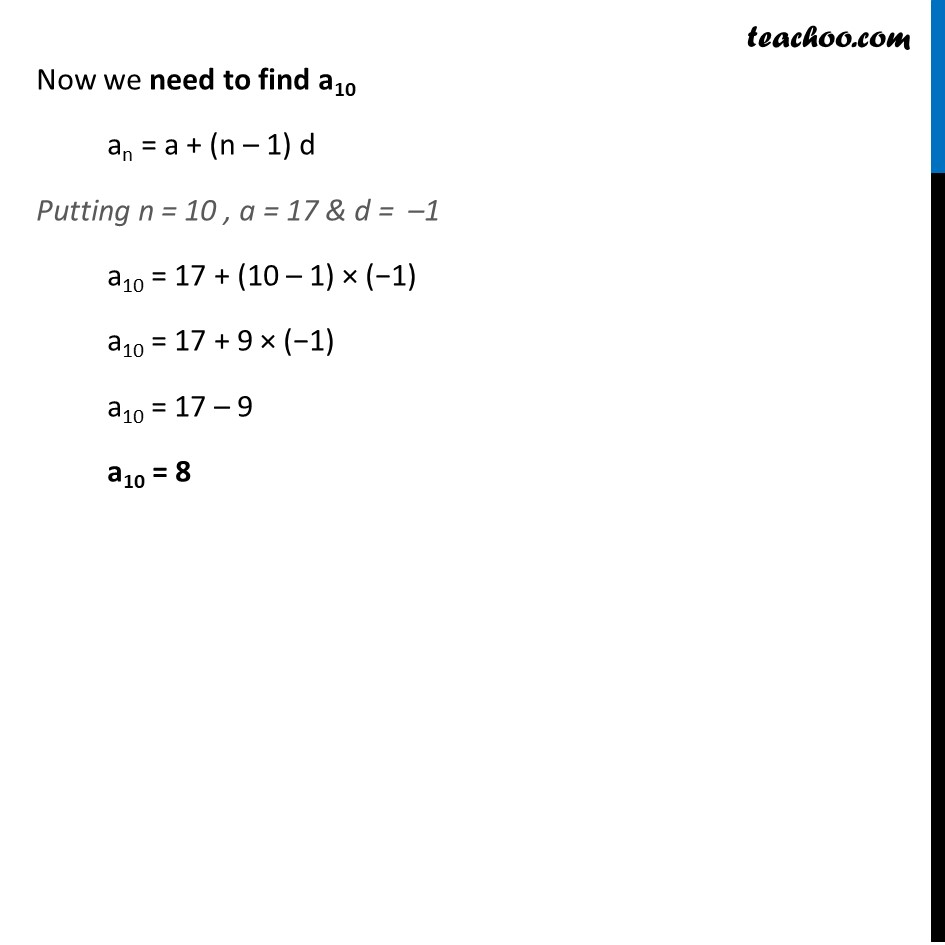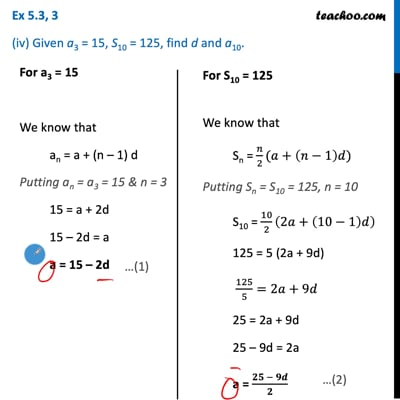Ex 5.3

Chapter 5 Class 10 Arithmetic Progressions (Term 2)
Serial order wiseThis video is only available for Teachoo black users

### Transcript

Ex 5.3, 3 (iv) Given a3 = 15, S10 = 125, find d and a10. For a3 = 15 We know that an = a + (n – 1) d Putting an = a3 = 15 & n = 3 15 = a + 2d 15 – 2d = a a = 15 – 2d For S10 = 125 We know that Sn = 𝑛/2(𝑎+(𝑛−1)𝑑) Putting Sn = S10 = 125, n = 10 S10 = 10/2 (2𝑎+(10−1)𝑑) 125 = 5 (2a + 9d) 125/5=2𝑎+9𝑑 25 = 2a + 9d 25 – 9d = 2a a = (𝟐𝟓 − 𝟗𝒅)/𝟐 From (1) and (2) 15 – 2d = (𝟐𝟑−𝟗𝒅)/𝟐 30 – 4d = 25 – 9d 5 = – 5d 5/(−5)=𝑑 d = –1 Putting value of d in (1) a = 15 – 2d a = 15 – 2 ×(−1) a = 15 + 2 a = 17 Now we need to find a10 an = a + (n – 1) d Putting n = 10 , a = 17 & d = –1 a10 = 17 + (10 – 1) × (−1) a10 = 17 + 9 × (−1) a10 = 17 – 9 a10 = 8